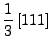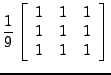Next: spectral Up: Implementation Previous: Implementation   Contents

## spatialconv

The complex interferogram is convoluted with a kernel by FFT's. The card PF_KERNEL specifies the 1D kernel. The 2D kernel is computed as: PF_KERNELPF_KERNEL, e.g. for a 3 point moving average 1D kernel(1)

This becomes(2)

The blocksize for the convolution is chosen as high as possible. A 2D kernel can be specified in an input file. Only odd sized kernels can be used, but simply add a zero to an odd kernel.

If a real4 matrix containing phase should be convoluted by a certain kernel, first convert this real4 to a complex real4 matrix. Do this either by computing the phase for complex umbers with amplitude 1, or by setting the real part of the file to the phase and the imaginary part to 1 (arbitrary).

Leijen 2009-04-14